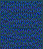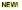# Car Audio Equations

Here's a summary of all of the equations used throughout this site. If we miss any please let us know.

## Ohm's Law

E=I*R

Where

E = voltage in volts
I = current in amps
R = resistance in ohms

Power equation

P = E*I

Parallel Resistances

1/Rt = 1/R1 + 1/R2 + 1/R3...

Where

Rt = total resistance

R1-R3 = individual resistances

Series Resistances

Rt = R1 + R2 + R3...

Where

Rt = total resistance

R1-R3 = individual resistances

## Wavelength

wavelength = (speed of sound)/(frequency)

wavelength = feet

speed of sound = feet per second

frequency = Hertz

## SPL Calculation

10 x log10(Power) + Efficiency = Maximum Output

Power = watts

Efficiency = decibels

Maximum Output = decibels

## Conversions

Kilo = 1,000

Mega = 1,000,000

Milli = 1/1,000

Micro = 1/1,000,000

1 cubic foot = 1,728 cubic inchesWeb caraudiobook.comTraining DVDsComplete DVD Special
Car Stereo Installation
Car Alarm Installation
Fiberglass Fabrication
Fiberglass Fabrication IICar Audio Installation
Installation Goodies
Installations You Can Do
Car Stereo HelpCredits
Equations
Contact